Question

# Consider the chemical reaction 2NH3(g) ó N2(g) + 3H2(g). The equilibrium is to be established in...

Consider the chemical reaction 2NH3(g) ó N2(g) + 3H2(g). The equilibrium is to be established in a 50.0 L container at 1,000 K, where Kc = 4.0 × 10-2. Initially, 6.10 x 105 moles of NH3(g) are present. Calculate the amount of H2 presentat equilibrium. [H2] =_____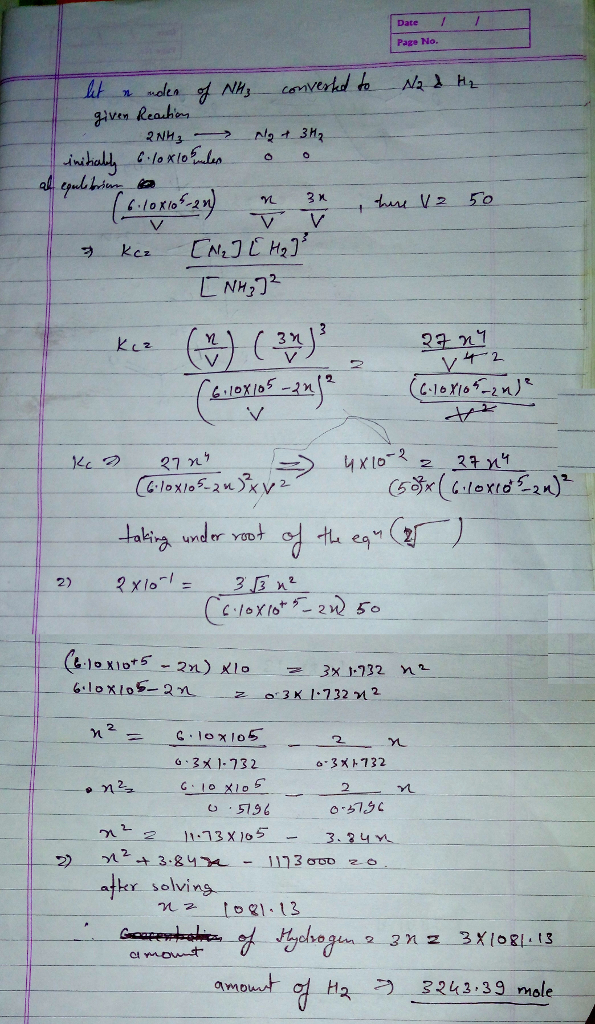#### Earn Coins

Coins can be redeemed for fabulous gifts.

Similar Homework Help Questions
• ### The equilibrium constant, Kc, for the following reaction is 6.30 at 723K. 2NH3(g) N2(g) + 3H2(g)...

The equilibrium constant, Kc, for the following reaction is 6.30 at 723K. 2NH3(g) N2(g) + 3H2(g) If an equilibrium mixture of the three gases in a 15.7 L container at 723K contains 0.284 mol of NH3(g) and 0.437 mol of N2, the equilibrium concentration of H2 is__________ M.

• ### Consider the following reaction where K = 0.159 at 723 K: N2(g) + 3H2(g) = 2NH3(g)...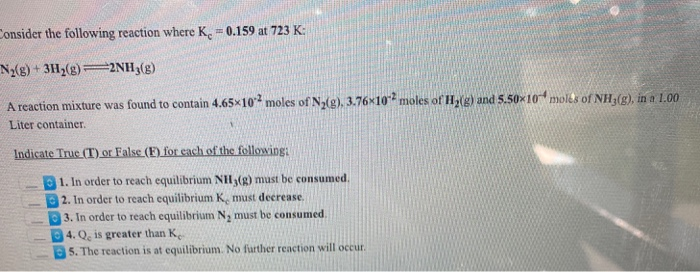Consider the following reaction where K = 0.159 at 723 K: N2(g) + 3H2(g) = 2NH3(g) A reaction mixture was found to contain 4.65*10*2 moles of N2(g), 3.76x10-2 moles of H2(g) and 5.50*10-4 moles of NH3(g), in a 1.00 Liter container Indicate Truc (T) or False (E) for each of the followingi 1. In order to reach equilibrium NH3(g) must be consumed. 2. In order to reach equilibrium K must decrease. 3. In order to reach equilibrium N, must be...

• ### Consider the following reaction: N2(g)+3H2(g)⇌2NH3(g) Complete the following table. Assume that all concentrations are equilibrium concentrations...

Consider the following reaction: N2(g)+3H2(g)⇌2NH3(g) Complete the following table. Assume that all concentrations are equilibrium concentrations in M. T (K) [N2] [H2] [NH3] [Kc] 500 0.115 0.105 0.439 − 575 0.105 M − 0.128 M 9.6 775 0.130 M 0.145 M − 0.0584 Find Kc at 500 K. Find [H2] at 575 K. Find [NH3] at 775 K.

• ### Consider the following reaction: 2NH3(8) N2(g) + 3H2(g) If 4.23x10 moles of NH3, 0.213 moles of Nz, and 0.272 moles...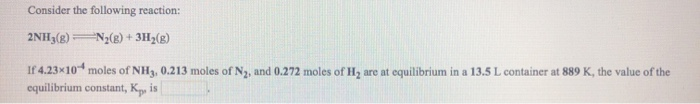Consider the following reaction: 2NH3(8) N2(g) + 3H2(g) If 4.23x10 moles of NH3, 0.213 moles of Nz, and 0.272 moles of H, are at equilibrium in a 13.5 L container at 889 K, the value of the equilibrium constant, Ky, is

• ### Consider the following reaction where K. = 6.30 at 723 K. 2NH3(B) = N2(g) + 3H2() A reaction mixture was found to c...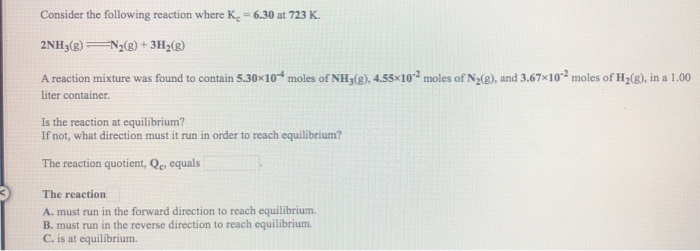Consider the following reaction where K. = 6.30 at 723 K. 2NH3(B) = N2(g) + 3H2() A reaction mixture was found to contain 5.30*10* moles of NH3(g), 4.55*10moles of N2(8), and 3.67x10-2 moles of H2(e), in a 1.00 liter container Is the reaction at equilibrium? If not, what direction must it run in order to reach equilibrium? The reaction quotient, Qc, equals The reaction A. must run in the forward direction to reach equilibrium B. must run in the reverse...

• ### A student ran the following reaction in the laboratory at 691 K: N2(g) + 3H2(g) 2NH3(g)...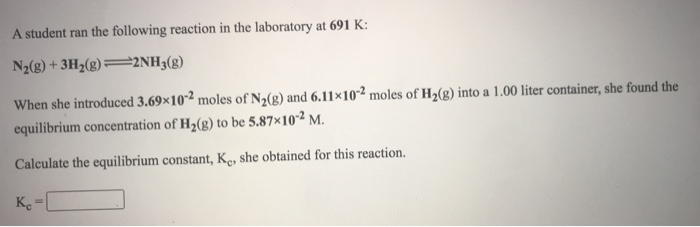A student ran the following reaction in the laboratory at 691 K: N2(g) + 3H2(g) 2NH3(g) When she introduced 3.69x10-2 moles of N2(g) and 6.11x10-2 moles of H2(g) into a 1.00 liter container, she found the equilibrium concentration of H2(8) to be 5.87*10-2 M. Calculate the equilibrium constant, Kc, she obtained for this reaction. Ko

• ### A student ran the following reaction in the laboratory at 745 K: N2(g) + 3H2(g) 2NH3(g)...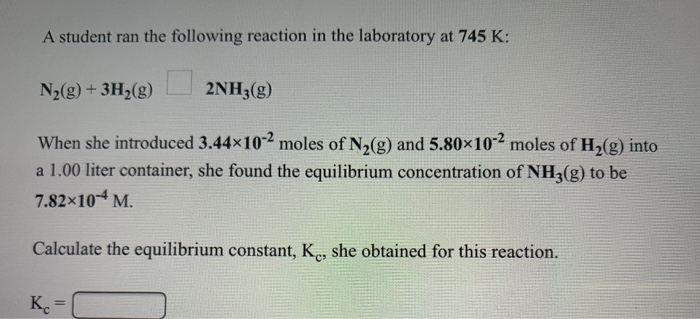A student ran the following reaction in the laboratory at 745 K: N2(g) + 3H2(g) 2NH3(g) When she introduced 3.44x10-2 moles of N2(g) and 5.80x10-2 moles of H2(g) into a 1.00 liter container, she found the equilibrium concentration of NH3(g) to be 7.82x10-4M. Calculate the equilibrium constant, K., she obtained for this reaction. K=

• ### Calculate KC in terms of molar concentration for the reaction N2(g) + 3H2(g)   2NH3(g) when the...

Calculate KC in terms of molar concentration for the reaction N2(g) + 3H2(g)   2NH3(g) when the equilibrium concentration moles per liter are: N2 = 0.02, H2 = 0.01, NH3 = 0.10.

• ### For the reaction N2(g)+3H2(g)⇌2NH3(g) what is the value of Kc at 500 ∘C if the equilibrium...

For the reaction N2(g)+3H2(g)⇌2NH3(g) what is the value of Kc at 500 ∘C if the equilibrium concentrations are as follows: [H2] = 0.45 M , [N2] = 0.50 M , and [NH3] = 2.0 M ?

• ### Consider the following reaction where K. = 6.30 at 723 K. 2NH3() N2(g) + 3H2(8) A...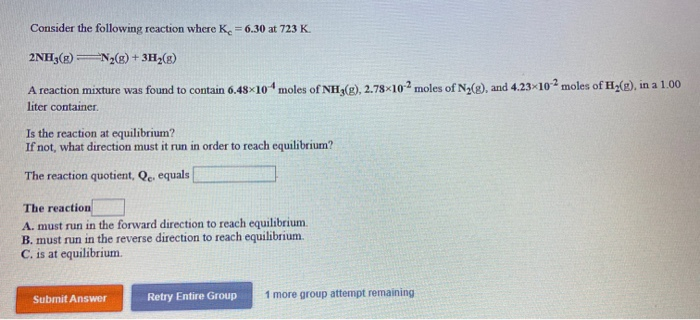Consider the following reaction where K. = 6.30 at 723 K. 2NH3() N2(g) + 3H2(8) A reaction mixture was found to contain 6.48*10* moles of NH3(g), 2.78 102 moles of N2(e), and 4.23-102 moles of H2(g), in a 1.00 liter container Is the reaction at equilibrium? If not, what direction must it run in order to reach equilibrium? The reaction quotient, Qc equals The reaction A. must run in the forward direction to reach equilibrium. B. must run in the...